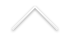Título: 2D anisotropic KPZ at stationarity

Palestrante: Dirk Erhard (IM- UFBA)
Data: 19/10/2020
Horário: 15:00 (Rio de Janeiro)
Local: Transmissão online.

Confira AQUI o link para a transmissão

Resumo: The KPZ equation is the stochastic partial differential equation in d space dimensions formally given by \partial_t h=\Delta h +\langle h,Q h\rangle +\xi, where \xi is the so called space time white noise, i.e., a gaussian process with short range correlations, and Q is a d dimensional matrix. This equation was introduced in the physics literature in the late eighties to model stochastic growth phenomena, is moreover connected to (d+1) dimensional directed polymers in a random potential and is supposed to arise as a scaling limit of a large class of interacting particle systems.
In this talk I will try to explain where this equation comes from, why it is interesting, and how its behaviour depends on the spatial
dimension. I will mostly focus on the case of dimension 2, and I will comment on a recent result which contradicts a folklore belief from the physics literature.

This is based on joint works with Giuseppe Cannizzaro, Philipp Schönbauer and Fabio Toninelli.